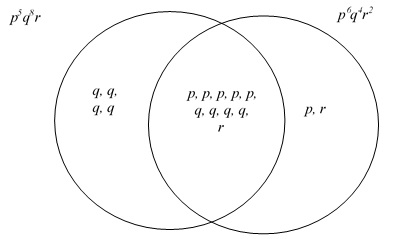## 1.

The Correct Answer is (A) — When we divide each side of the inequality by 50, we get x>-0.4. This means that we want all the values that are greater than -0.4. Since x cannot equal -0.4, answer choice (A) is correct.

## 2.

The Correct Answer is (B) — Since one lap around the track is 400m and John runs three laps, the total distance John runs is 400m × 3 = 1200m. He runs this distance in ten minutes, so his speed is 1200m/(10 min) = 120 m/min. Converting this to meters per second gives us (120 m/min)(min/(60 sec))=2 m/s.

## 3.

The Correct Answer is (C) — Multiplying each side of the equation by 50 gives us 50(m - 2) = 50x/5 which simplifies to 10x = 50m - 100.

## 4.

The Correct Answer is (B) — From the chart, we can observe that every time x increases by 5, f(x) decreases by 10. This would mean that f(25)=40, and then f(30)=30.

## 5.

The Correct Answer is (C) — After the first month, Armin has $20,000 -$2000 = $18,000 remaining. Since each subsequent month costs$400, he can lease the car for an additional $18,000/$400 = 45. These 45 months plus the first month equals 46 months in total.

## 6.

The Correct Answer is (B) — Adding the two equations gives us 3x + 3y = 24. Dividing each side of this equation by 3 gives us x + y = 8.

## 10.

The Correct Answer is (A) — We can recognize that is a difference of squares and factor the equation into y = (x+6)(x-6). Solving for the roots gives us x = 6 and x = -6. The question states that a > b so a must equal 6 and b must equal -6. a/b gives us 6/(-6) = -1.

## 11.

The Correct Answer is (D) — There are 250 bacteria when t = 0, so this eliminates answer choice (A). The colony doubles twice per day, and so the exponent must be 2t. This gives us answer choice (D).

## 12.

The Correct Answer is (B) — Squaring both sides of the equation gives us . Multiplying out the right side gives us . Moving all the terms to the right side gives us . Factoring the equation gives us . The only value possible for is 3, so the correct answer is (B).

## 13.

The Correct Answer is (A) — The equation for a circle is where (a,b) is the center of the circle and r is the radius of the circle. Since the circle in the question has a radius of 6 and a center of (-3,7), the equation is .

## 14.

The Correct Answer is (C) — To solve this problem, we can consider which factors the two terms share and which they don’t. A Venn diagram of this would look like the following:Multiplying all the factors shown above, counting the shared ones only once, we get .

## 15.

The Correct Answer is (B) — The distance a point on the outer edge of the wheel travels in one rotation is equal to the circumference of the wheel. Since the diameter is 50m, the circumference is C = dπ = 50π meters. Dividing this by the 3 minutes it takes the wheel to make one rotation, we get (50π meters)/(3 min). Converting this to meters per second gives us ((50π meters)/(3 min))(min/(60 sec)) = 5π/(18 ) meters per second.

## 16.

The Correct Answer is ((2&lt;3x&lt;5)) — Multiplying all parts of the inequality by 3 gives us 3 < 3x + 1 < 6. Subtracting 1 from all parts of the inequality gives us 2 < 3x < 5. Any value of 3x that fits into this inequality is a valid answer.

## 17.

The Correct Answer is (6) — The length of this triangular prism is represented as x. The area of the triangular base is found by (4 × 3)/2 = 6. We are told that the volume is equal to the product of the length and the area of the face. We are also told that the volume is 36, which gives us 6x = 36. Solving for x gives us x = 6.

## 18.

The Correct Answer is ((2/3 or 0.66 or 0.67)) — We are told that line n is perpendicular to the line y = 3x - 1. This means that line n must have a slope of -1/3. A slope of -1/3 indicates that for each unit decrease in x, y increases by 1/3. We are given that one of the points on line n is (2, 0). Using the slope, we know that when x = 1, the point must be (1,1/3). Tracing back one more step, when x = 0, the point must be (0,2/3).

## 19.

The Correct Answer is ((4)) — When we set the height, which is described by h(t), to 1.5, we get . Subtracting 1.5 from each side gives us . Factoring the right side gives us . Setting equal to 0 gives us , and solving for gives us .

## 20.

The Correct Answer is (5) — Multiplying each side of the equation by gives us. Distributing all the parentheses on both sides gives us . Combining like terms on the left gives us , and multiplying both sides by 2 gives us . Distributing in the 5 on the right side gives us , and moving all the terms to the right side gives us. Factoring gives us, meaning that solutions to this equation are and . Since the problem tells us that is a positive number, the answer is 5.